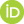# Axiomatization of Galilean Spacetime

Ketland, Jeffrey (2023) Axiomatization of Galilean Spacetime. [Preprint]Text Gal_Pitt_Mar_2023.pdf Download (905kB)

## Abstract

In this article, we give a second-order synthetic axiomatization $\Gal(1,3)$ for Galilean spacetime, the background spacetime of Newtonian classical mechanics. The primitive notions of this theory are the 3-place predicate of betweenness $\bet$, the 2-place predicate of simultaneity $\sim$ and a 4-place congruence predicate, written $\equiv^{\sim}$, restricted to simultaneity hypersurfaces. We define a standard coordinate structure $\G^{(1,3)}$, whose carrier set is $\R^4$, and which carries relations (on $\R^4$) corresponding to $\bet$, $\sim$ and $\equiv^{\sim}$. This is the standard model of $\Gal(1,3)$. We prove that the symmetry group of $\G^{(1,3)}$ is the (extended) Galilean group (an extension of the usual 10-parameter Galilean group, with two additional parameters for length and time scalings). We prove that each full model of $\Gal(1,3)$ is isomorphic to $\G^{(1,3)}$.

 Export/Citation: EndNote | BibTeX | Dublin Core | ASCII/Text Citation (Chicago) | HTML Citation | OpenURL
 Social Networking:

Item Type: Preprint
Creators:
CreatorsEmailORCIDKetland, Jeffreyjeffreyketland@gmail.com0000-0002-5128-4387
Keywords: Galilean spacetime, Representation theorem, Geometry
Subjects: Specific Sciences > Physics > Classical Physics
Depositing User: Dr Jeffrey Ketland
Date Deposited: 01 Mar 2023 15:40
Item ID: 21809
Subjects: Specific Sciences > Physics > Classical Physics
Date: 1 March 2023
URI: http://philsci-archive.pitt.edu/id/eprint/21809

### Monthly Views for the past 3 yearsView Item